Updating search results...

# 22 Results

View
Selected filters:
• patterns-and-equations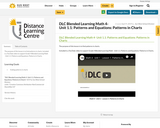Conditional Remix & Share Permitted
CC BY-NC-SA
Rating
0.0 stars

The purpose of this lesson is to find patterns in charts.

Included is a YouTube video to support Grade 4 Blended Learning Math - Unit 1.1: Patterns and Equations: Patterns in Charts.

Subject:
Math
Material Type:
Activity/Lab
Homework/Assignment
Lesson
Provider:
Sun West Distance Learning Centre (DLC)
04/09/2019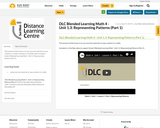Conditional Remix & Share Permitted
CC BY-NC-SA
Rating
0.0 stars

The purpose of this lesson is to use concrete materials to copy a pattern in a table.

Included is a YouTube video to support Grade 4 Blended Learning Math - Unit 1.3: Representing Patterns (Part 1).

Subject:
Math
Material Type:
Activity/Lab
Homework/Assignment
Lesson
Provider:
Sun West Distance Learning Centre (DLC)
04/09/2019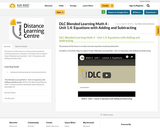Conditional Remix & Share Permitted
CC BY-NC-SA
Rating
0.0 stars

The purpose of this lesson is to make and solve equations involving subtraction.

Included is a YouTube video to support Grade 4 Blended Learning Math - Unit 1.4: Equations with Adding and Subtracting.

Subject:
Math
Material Type:
Activity/Lab
Homework/Assignment
Lesson
Provider:
Sun West Distance Learning Centre (DLC)
04/09/2019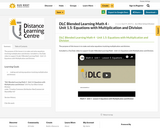Conditional Remix & Share Permitted
CC BY-NC-SA
Rating
0.0 stars

The purpose of this lesson is to make and solve equations involving multiplication and division.

Included is a YouTube video to support Grade 4 Blended Learning Math - Unit 1.5: Equations with Multiplication and Division.

Subject:
Math
Material Type:
Activity/Lab
Homework/Assignment
Lesson
Provider:
Sun West Distance Learning Centre (DLC)
04/09/2019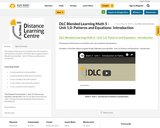Conditional Remix & Share Permitted
CC BY-NC-SA
Rating
0.0 stars

The purpose of this lesson is to introduce the unit on patterns and equations.

Included is a YouTube video to support Grade 5 Blended Learning Math - Unit 1.0: Patterns and Equations - Introduction.

Subject:
Math
Material Type:
Activity/Lab
Homework/Assignment
Lesson
Provider:
Sun West Distance Learning Centre (DLC)
05/10/2019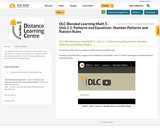Conditional Remix & Share Permitted
CC BY-NC-SA
Rating
0.0 stars

The purpose of this lesson is to explore number patterns and pattern rules.

Included is a YouTube video to support Grade 5 Blended Learning Math - Unit 1.1: Patterns and Equations- Number Patterns and Pattern Rules.

Subject:
Math
Material Type:
Activity/Lab
Homework/Assignment
Lesson
Provider:
Sun West Distance Learning Centre (DLC)
05/10/2019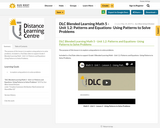Conditional Remix & Share Permitted
CC BY-NC-SA
Rating
0.0 stars

The purpose of this lesson is to explore using patterns to solve problems.

Included is a YouTube video to support Grade 5 Blended Learning Math - Unit 1.2: Patterns and Equations- Using Patterns to Solve Problems.

Subject:
Math
Material Type:
Activity/Lab
Homework/Assignment
Lesson
Provider:
Sun West Distance Learning Centre (DLC)
05/10/2019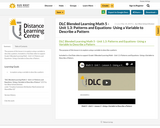Conditional Remix & Share Permitted
CC BY-NC-SA
Rating
0.0 stars

The purpose of this lesson is to explore using a variable to describe a pattern.

Included is a YouTube video to support Grade 5 Blended Learning Math - Unit 1.3: Patterns and Equations- Using a Variable to Describe a Pattern.

Subject:
Math
Material Type:
Activity/Lab
Homework/Assignment
Lesson
Provider:
Sun West Distance Learning Centre (DLC)
05/10/2019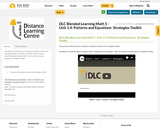Conditional Remix & Share Permitted
CC BY-NC-SA
Rating
0.0 stars

The purpose of this lesson is to explore strategies to add to the strategies toolkit.

Included is a YouTube video to support Grade 5 Blended Learning Math - Unit 1.4: Patterns and Equations- Strategies Toolkit.

Subject:
Math
Material Type:
Activity/Lab
Homework/Assignment
Lesson
Provider:
Sun West Distance Learning Centre (DLC)
05/10/2019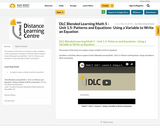Conditional Remix & Share Permitted
CC BY-NC-SA
Rating
0.0 stars

The purpose of this lesson is to explore using a variable to write an equations.

Included is a YouTube video to support Grade 5 Blended Learning Math - Unit 1.5: Patterns and Equations- Using a Variable to Write an Equation.

Subject:
Math
Material Type:
Activity/Lab
Homework/Assignment
Lesson
Provider:
Sun West Distance Learning Centre (DLC)
05/10/2019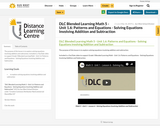Conditional Remix & Share Permitted
CC BY-NC-SA
Rating
0.0 stars

The purpose of this lesson is to explore solving equations involving addition and subtraction.

Included is a YouTube video to support Grade 5 Blended Learning Math - Unit 1.6: Patterns and Equations - Solving Equations Involving Addition and Subtraction.

Subject:
Math
Material Type:
Activity/Lab
Homework/Assignment
Lesson
Provider:
Sun West Distance Learning Centre (DLC)
05/10/2019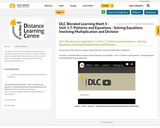Conditional Remix & Share Permitted
CC BY-NC-SA
Rating
0.0 stars

The purpose of this lesson is to explore solving equations involving multiplication and division.

Included is a YouTube video to support Grade 5 Blended Learning Math - Unit 1.7: Patterns and Equations - Solving Equations Involving Multiplication and Division.

Subject:
Math
Material Type:
Activity/Lab
Homework/Assignment
Lesson
Provider:
Sun West Distance Learning Centre (DLC)
05/10/2019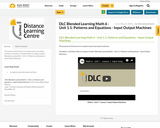Conditional Remix & Share Permitted
CC BY-NC-SA
Rating
0.0 stars

The purpose of this lesson is to explore input and output machines.

Included is a YouTube video to support Grade 6 Blended Learning Math - Unit 1.1: Patterns and Equations - Input Output Machines.

Subject:
Math
Material Type:
Activity/Lab
Lesson
Open Access Asset
Teaching/Learning Strategy
Provider:
Sun West Distance Learning Centre (DLC)
09/01/2023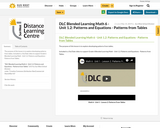Conditional Remix & Share Permitted
CC BY-NC-SA
Rating
0.0 stars

The purpose of this lesson is to explore developing patterns from tables.

Included is a YouTube video to support Grade 6 Blended Learning Math - Unit 1.2: Patterns and Equations - Patterns from Tables.

Subject:
Math
Material Type:
Activity/Lab
Homework/Assignment
Lesson
Provider:
Sun West Distance Learning Centre (DLC)
05/14/2019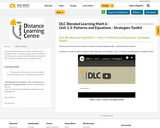Conditional Remix & Share Permitted
CC BY-NC-SA
Rating
0.0 stars

The purpose of this lesson is to add to a problem solving strategies toolkit - specifically using a pattern.

Included is a YouTube video to support Grade 6 Blended Learning Math - Unit 1.3: Patterns and Equations - Strategies Toolkit.

Subject:
Math
Material Type:
Activity/Lab
Homework/Assignment
Lesson
Provider:
Sun West Distance Learning Centre (DLC)
05/14/2019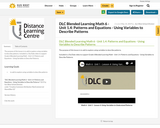Conditional Remix & Share Permitted
CC BY-NC-SA
Rating
0.0 stars

The purpose of this lesson is to add to explore using variables to describe patterns.

Included is a YouTube video to support Grade 6 Blended Learning Math - Unit 1.4: Patterns and Equations - Using Variables to Describe Patterns.

Subject:
Math
Material Type:
Activity/Lab
Homework/Assignment
Lesson
Provider:
Sun West Distance Learning Centre (DLC)
05/14/2019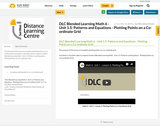Conditional Remix & Share Permitted
CC BY-NC-SA
Rating
0.0 stars

The purpose of this lesson is to explore plotting points on a co-ordinate grid.

Included is a YouTube video to support Grade 6 Blended Learning Math - Unit 1.5: Patterns and Equations - Plotting Points on a Co-ordinate Grid.

Subject:
Math
Material Type:
Activity/Lab
Assessment
Lesson
Provider:
Sun West Distance Learning Centre (DLC)
05/14/2019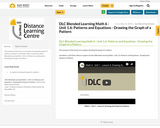Conditional Remix & Share Permitted
CC BY-NC-SA
Rating
0.0 stars

The purpose of this lesson is to explore drawing the graph of a pattern.

Included is a YouTube video to support Grade 6 Blended Learning Math - Unit 1.6: Patterns and Equations - Drawing the Graph of a Pattern.

Subject:
Math
Material Type:
Activity/Lab
Homework/Assignment
Lesson
Provider:
Sun West Distance Learning Centre (DLC)
05/14/2019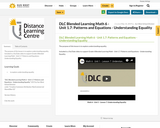Conditional Remix & Share Permitted
CC BY-NC-SA
Rating
0.0 stars

The purpose of this lesson is to explore understanding equality.

Included is a YouTube video to support Grade 6 Blended Learning Math - Unit 1.7: Patterns and Equations - Understanding Equality.

Subject:
Math
Material Type:
Activity/Lab
Homework/Assignment
Lesson
Provider:
Sun West Distance Learning Centre (DLC)
05/14/2019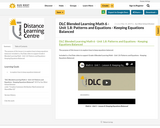Conditional Remix & Share Permitted
CC BY-NC-SA
Rating
0.0 stars

The purpose of this lesson is to explore how to keep equations balanced.

Included is a YouTube video to support Grade 6 Blended Learning Math - Unit 1.8: Patterns and Equations - Keeping Equations Balanced.

Subject:
Math
Material Type:
Activity/Lab
Homework/Assignment
Lesson
Provider:
Sun West Distance Learning Centre (DLC)